# Money Back GuaranteeGet LifePak®+
Rewards (ADR) order and watch your
Skin Carotenoid Score increase with
the BioPhotonic Scanner!

The Scanner is here to help you see how taking the Scanner certified products regularly increase your SCS. If after taking the products for 90 days your SCS does not increase, your money will be refunded*.

*Below is the Money Back Guarantee terms and conditions along with the Claims Form.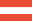PDF(DE) PDF(EN)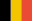PDF(NL) PDF(FR) PDF(EN)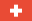PDF(DE) PDF(FR) PDF(IT) PDF(EN)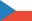PDF(CZ) PDF(EN)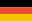PDF(DE) PDF(EN)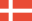PDF(DK) PDF(EN)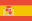PDF(ES) PDF(EN)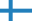PDF(EN) PDF(FI)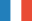PDF(FR) PDF(EN)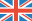PDF(EN)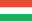PDF(HU) PDF(EN)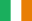PDF(EN)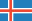PDF(IS) PDF(EN)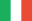PDF(IT) PDF(EN)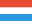PDF(DE) PDF(FR) PDF(EN)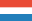PDF(NL) PDF(EN)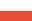PDF(PL) PDF(EN)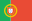PDF(EN) PDF(PT)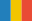PDF(RO) PDF(EN)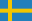PDF(SE) PDF(EN)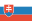PDF(SK) PDF(EN)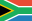PDF(EN)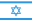PDF(EN)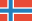PDF(NO) PDF(EN)